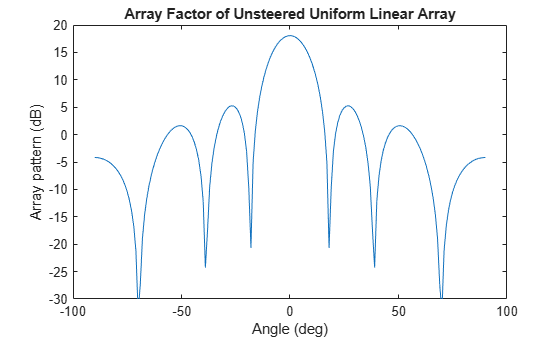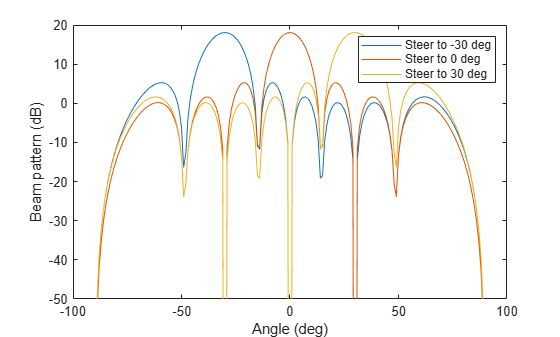# arrayfactor

Array factor of sensor arrays

## Syntax

``af = arrayfactor(pos,ang)``
``af = arrayfactor(pos,ang,wts)``

## Description

example

````af = arrayfactor(pos,ang)` returns the array factor `af` for an array of elements located at positions `pos` and arrival directions `ang`.```

example

````af = arrayfactor(pos,ang,wts)` also specifies array steering weights `wts`.```

## Examples

collapse all

Compute the array factor for an unsteered 8-element ULA operating at 100 MHz. Elements are placed 0.4 wavelengths apart.

```freq = 100e6; c = physconst('LightSpeed'); lam = c/freq; pos = (0:7)*0.4; ang = -90:90; af = arrayfactor(pos,ang); plot(ang,mag2db(abs(af.'))) xlabel('Angle (deg)') ylabel('Array pattern (dB)') ylim([-30 20]) title('Array Factor of Unsteered Uniform Linear Array')```Display the array beam patterns for an 8-element ULA with half-wavelength spacing. Steer the array to -30, 0, and 30 degrees.

Set the positions of the ULA elements.

`pos = (0:7)*0.5;`

Define the steering direction weights.

```angsteer = [-30 0 30]; wsteer = steervec(pos,angsteer);```

Compute the array factors for each steering direction for all angles.

```ang = -90:90; af = arrayfactor(pos,ang,wsteer);```

Plot the array factor for each steering direction.

```plot(ang,mag2db(abs(af.'))) xlabel('Angle (deg)') ylabel('Beam pattern (dB)') ylim([-50 20]) legend('Steer to -30 deg','Steer to 0 deg','Steer to 30 deg')```## Input Arguments

collapse all

Positions of the elements of a sensor array specified as a 1-by-N vector, a 2-by-N matrix, or a 3-by-N matrix. In this vector or matrix, N represents the number of elements of the array. Each column of `pos` represents the coordinates of an element. If `pos` is a 1-by-N vector, then it represents the y-coordinate of the sensor elements of a line array. The x and z-coordinates are assumed to be zero. When `pos` is a 2-by-N matrix, it represents the (y,z)-coordinates of the sensor elements of a planar array. This array is assumed to lie in the yz-plane. The x-coordinates are assumed to be zero. When `pos` is a 3-by-N matrix, then the array can have an arbitrary shape. Sensor positions are in terms of signal wavelength.

Example: `[0,0,0; 0.1,0.4,0.3; 1,1,1]`

Data Types: `double`

Arrival directions of incoming signals specified as a 1-by-M vector or a 2-by-M matrix, where M is the number of incoming signals. If `ang` is a 2-by-M matrix, each column specifies the direction in azimuth and elevation of the incoming signal `[az;el]`. The azimuth angle must lie between –180° and 180° and the elevation angle must lie between –90° and 90°. The azimuth angle is the angle between the x-axis and the projection of the arrival direction vector onto the xy plane. It is positive when measured from the x-axis toward the y-axis. The elevation angle is the angle between the arrival direction vector and xy-plane. It is positive when measured towards the z axis. If `ang` is a 1-by-M vector, then it represents a set of azimuth angles with the elevation angles assumed to be zero. Angle units are specified in degrees.

Example: [45;0]

Data Types: `double`

Array weights, specified as a complex-valued N-by-1 vector or complex-valued N-by-L matrix. Each column of `wts` corresponds to a different set of weights for the array. L is the number of weight sets.

Data Types: `double`
Complex Number Support: Yes

## Output Arguments

collapse all

Array factor of sensor array, returned as a 1-by-M complex-valued vector or L-by-M complex-valued matrix. Each column contains the array factor for a different set of weights `wts`. Each row corresponds to one of the angles specified in `ang`.

 Van Trees, Harry L. Detection, Estimation, and Modulation Theory. 4: Optimum Array Processing. Wiley, 2002.

 Johnson, Don H. and D. Dudgeon. Array Signal Processing. Englewood Cliffs, NJ: Prentice Hall, 1993.

 Van Veen, B.D. and K. M. Buckley. “Beamforming: A versatile approach to spatial filtering”